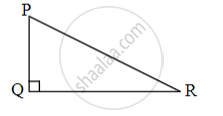# Choose the correct alternative: In right angled triangle, if sum of the squares of the sides of right angle is 169, then what is the length of the hypotenuse? - Geometry

MCQ

Choose the correct alternative:

In right angled triangle, if sum of the squares of the sides of right angle is 169, then what is the length of the hypotenuse?

• 15

• 13

• 5

• 12

#### Solution

13In ∆PQR,

∠Q = 90°

∴ PR2 = PQ2 + QR2    ...[Pythagoras theorem]

∴ PR2 = 169               ...[Given]

∴ PR = sqrt(169)

Taking square root both the sides,

∴ PR = 13

Concept: Converse of Pythagoras Theorem
Is there an error in this question or solution?

Share